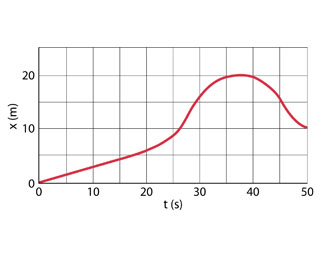Question
3 views

The position of a rabbit along a straight tunnel as a function of time is plotted in the figure.

What is its instantaneous velocity at t=10.0s?

What is its instantaneous velocity at t=30.0s?

What is its average velocity between t=0 and t=5.0s?

What is its average velocity between t=25.0s and t=30.0s?

What is its average velocity between t=40.0s and t=50.0s?help_outlineImage Transcriptionclose40 10 30 t(s) 50 20 20 (u) x fullscreen
check_circle

Step 1

Draw a tangent line at t=10 s and t=30 s on the given plot that represents the position of the rabbit.

Step 2

Consider the tangent line at t=10 s from the above graph. The slope is less steep of the two drawn blue lines. The instantaneous velocity of the rabbit at t=10 s can be calculated from the slope as,

Step 3

Consider the tangent line at t=30 s from the above graph. The slope is steeper of the two drawn blue lines. It rises 25 ...

### Want to see the full answer?

See Solution

#### Want to see this answer and more?

Solutions are written by subject experts who are available 24/7. Questions are typically answered within 1 hour.*

See Solution
*Response times may vary by subject and question.
Tagged in

### Science